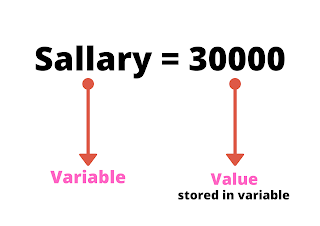What is a variable in Python?

Variables used to store data values. In other words, variables are reserved memory locations for storing values. This means that some space is reserved in your memory when you create a variable.

According to the data type of the variable, the interpreter allocates memory and decides what can be stored in the reserved memory. So by assigning different data types to variables, you can store integers, letters, or decimals in these variables.

Creating Variables:-

A variable is created when you assign a value to it and when you assign a value to a variable, it automatically reserves memory space. the equal mark i.e(=) is used to assign values to variables.

The operand to the left of the equal sign (=) operator is the name of the variable and the operand to the right of the equal sign (=) operator is the value stored in the variable.
For example −
A=10;
here, A is a Variable, and 10 is a value stored in the variable.
Another example is shown in the image below:-Variable Names

A variable can have a short name (like A and B) or a more descriptive name (Name, Roll_no, Age).
Rules for Python variables:
• The name of a variable must begin with a letter or an underscore character.
• Variable names contain alpha-numeric characters and underscores (A-z, 0-9 and _)
• Variable names are the case-sensitive(name, Name, and NAME are three different variables)

Legal variable names

myvar = "Technogyyan"
my_var = "Technogyyan"
_my_var = "Technogyyan"
myVar = "Technogyyan"
MYVAR = "Technogyyan"
myvar2 = "Technogyyan"

Illegal variable names

2myvar = "Technogyyan"
my-var = "Technogyyan"
my var = "Technogyyan"

These are some other example of variable:-
X=10
Y=10.5
Z="John"
a = 'John'
my_variable_name = "John"
b = str(3)
c= int(3)
d = float(3)
x, y, z = "Orange", "Banana", "Cherry"
x = y = z = "Orange"

In this image, you will find everything related to variables.You can check out the first 2 tutorials by clicking on the link below.